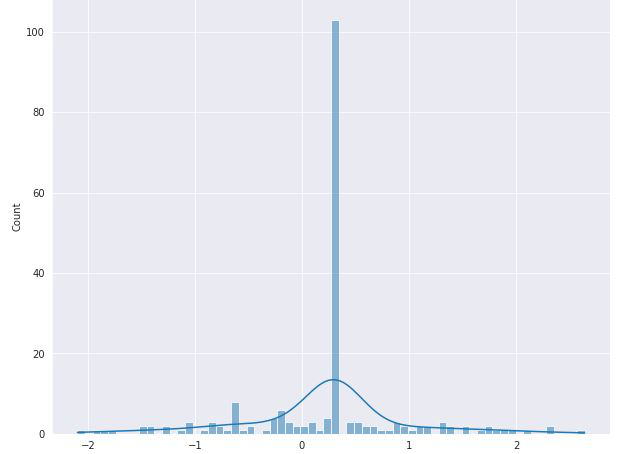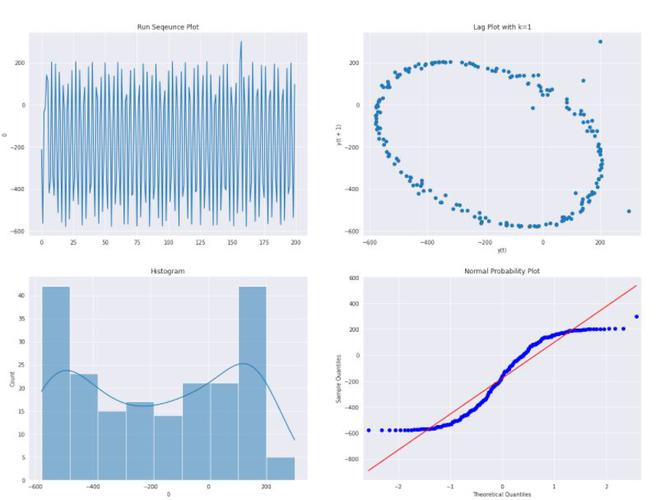Open in App
Not now

# Spectral Plot – Sinusoidal Model

• Last Updated : 31 Oct, 2022

The spectral plot is used to demonstrate the single dominant frequency of the plot. This indicates that the single cycle sinusoidal model might be appropriate.Consider the above plot, Let’s assume that the plot has distribution as follows:Now, we try to estimate the constant by the sample mean. This type of analysis would lead to an incorrect conclusion because:

• The sample mean is biased
• The confidence interval (CI) for the mean is too small.

Here, the proper choice of model is likewhere, a (alpha) is the amplitude, and w (omega) is the frequency of the observation and phi is the phase difference.

The next steps after this :

• Estimate the frequency of the spectral plot, this would be helpful in starting the value of subsequent non-linear fitting. We can use the Complex Demodulation phase plot to estimate it.
• Do a complex demodulation Amplitude plot to estimate the amplitude of the plot and to determine whether a constant amplitude is sufficient.
• Perform a non-linear fit of the model on the dataset.#### Implementation:

• In this implementation, we will be using a beam deflection case study, the dataset can be downloaded from here.

## Python3

 # necessary importsimport numpy as npimport pandas as pdimport matplotlib.pyplot as pltimport seaborn as snsimport statsmodels.api as sm%matplotlib inlinesns.mpl.rcParams['figure.figsize'] = (20.0, 15.0) # read beam databeam_data = pd.read_csv('beam_Deflection.txt', header=None) # draw 4 plotsns.set_style('darkgrid') fig, ax  = plt.subplots(2,2) sns.lineplot(x= pd.Series(beam_data.index),y =beam_data,ax =ax[0,0])ax[0,0].set_title('Run Sequence Plot') pd.plotting.lag_plot(beam_data,ax =ax[0,1])ax[0,1].set_title('Lag Plot with k=1') sns.histplot(beam_data,kde=True,ax =ax[1,0])ax[1,0].set_title('Histogram')sm.ProbPlot(beam_data).qqplot(line='s', ax=ax[1,1],color='blue');ax[1,1].set_title('Normal Probability Plot')  fig.suptitle('4-plot')plt.show()• The 4-plot(Lag plot) clearly shows that there is some periodic type of function that can be used to describe the dataset. Now, Let’s assume that the data can be fitted by the following equation:• Now, we plot the complex demodulation phase plot and complex demodulation amplitude plot to get the frequency and amplitude of the above equation. You can know more about how to plot complex demodulation phase and amplitude plot from here.
• The complex demodulation phase plot gives the frequency of 0.3025 and the complex demodulation amplitude plot gives the amplitude of around 390 after a cold start.

#### References:

My Personal Notes arrow_drop_up